# B29: Thin Lenses - Lens Equation, Optical Power

$$\newcommand{\vecs}{\overset { \rightharpoonup} {\mathbf{#1}} }$$ $$\newcommand{\vecd}{\overset{-\!-\!\rightharpoonup}{\vphantom{a}\smash {#1}}}$$$$\newcommand{\id}{\mathrm{id}}$$ $$\newcommand{\Span}{\mathrm{span}}$$ $$\newcommand{\kernel}{\mathrm{null}\,}$$ $$\newcommand{\range}{\mathrm{range}\,}$$ $$\newcommand{\RealPart}{\mathrm{Re}}$$ $$\newcommand{\ImaginaryPart}{\mathrm{Im}}$$ $$\newcommand{\Argument}{\mathrm{Arg}}$$ $$\newcommand{\norm}{\| #1 \|}$$ $$\newcommand{\inner}{\langle #1, #2 \rangle}$$ $$\newcommand{\Span}{\mathrm{span}}$$ $$\newcommand{\id}{\mathrm{id}}$$ $$\newcommand{\Span}{\mathrm{span}}$$ $$\newcommand{\kernel}{\mathrm{null}\,}$$ $$\newcommand{\range}{\mathrm{range}\,}$$ $$\newcommand{\RealPart}{\mathrm{Re}}$$ $$\newcommand{\ImaginaryPart}{\mathrm{Im}}$$ $$\newcommand{\Argument}{\mathrm{Arg}}$$ $$\newcommand{\norm}{\| #1 \|}$$ $$\newcommand{\inner}{\langle #1, #2 \rangle}$$ $$\newcommand{\Span}{\mathrm{span}}$$$$\newcommand{\AA}{\unicode[.8,0]{x212B}}$$

From the thin lens ray-tracing methods developed in the last chapter, we can derive algebraic expressions relating quantities such as object distance, focal length, image distance, and magnification.

Consider for instance the case of a converging lens with an object more distant from the plane of the lens than the focal point is. Here’s the diagram from the last chapter. In this copy, I have shaded two triangles in order to call your attention to them. Also, I have labeled the sides of those two triangles with their lengths.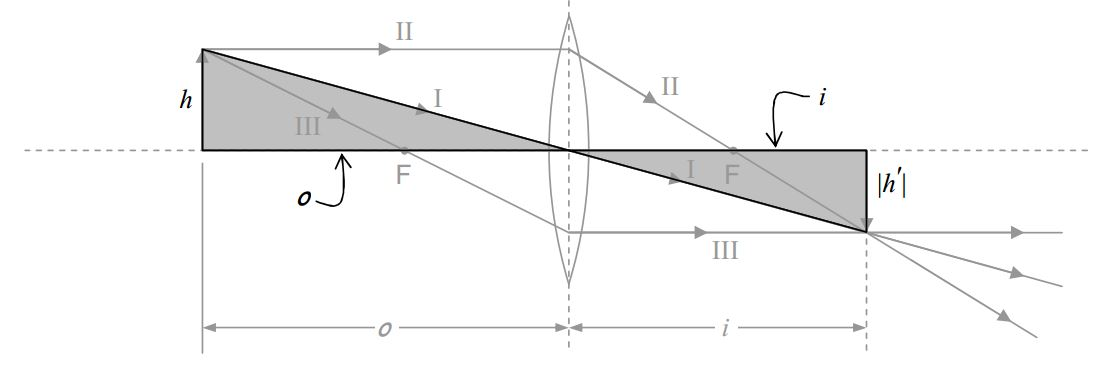By inspection, the two shaded triangles are similar to each other. As such, the ratios of corresponding sides are equal. Thus:

$\frac{|h'|}{h}=\frac{i}{o} \nonumber$

Recall the conventions stated in the last chapter:

Physical Quantity Symbol Sign Convention
focal length $$f$$ + for converging lens - for diverging lens
image distance $$i$$ + for real image - for virtual image
image height $$h'$$ + for erect image - for inverted image
magnification $$M$$ + for erect image - for inverted image

In the case at hand, we have an inverted image, so $$h'$$ is negative, so $$|h'|=-h'$$. Thus, the equation $$\frac{|h'|}{h}=\frac{i}{o}$$ can be written as $$\frac{-h'}{h}=\frac{i}{o}$$, or, as

$\frac{h'}{h}=-\frac{i}{o} \nonumber$

But $$\frac{h'}{h}$$ is, by definition, the magnification. Thus, we can write the magnification as:

$M=-\frac{i}{o} \label{29-1}$

Here’s another copy of the same diagram with another triangle shaded.By inspection, that shaded triangle is similar to the triangle that is shaded in the following copy of the same diagram:Using the fact that the ratios of corresponding sides of similar triangles are equal, we set the ratio of the two top sides (one from each triangle) equal to the ratio of the two right sides:

$\frac{o}{f}=\frac{h+|h'|}{|h'|} \nonumber$

Again, since the image is upside down, $$h'$$ is negative so $$|h'|=-h'$$. Thus

$\frac{o}{f}=\frac{h-h'}{-h'} \nonumber$

$\frac{o}{f}=1-\frac{h}{h'} \nonumber$

From our first pair of similar triangles we found that $$\frac{h'}{h}=-\frac{i}{o}$$ which can be written $$\frac{h}{h'}=-\frac{o}{i}$$ Substituting this into the expression $$\frac{o}{f}=1-\frac{h}{h'}$$ which we just found, we have

$\frac{o}{f}=1-\Big(-\frac{o}{i} \Big) \nonumber$

Dividing both sides by $$o$$ and simplifying yields:

$\frac{1}{f}=\frac{1}{o}+\frac{1}{i} \label{29-2}$

This equation is referred to as the lens equation. Together with our definition of the magnification $$M=\frac{h'}{h}$$, the expression we derived for the magnification $$M=-\frac{i}{o}$$, and our conventions:

Physical Quantity Symbol Sign Convention
focal length $$f$$ + for converging lens - for diverging lens
image distance $$i$$ + for real image - for virtual image
image height $$h'$$ + for erect image - for inverted image
magnification $$M$$ + for erect image - for inverted image

The lens equation tells us everything we need to know about the image of an object that is a known distance from the plane of a thin lens of known focal length. While we have derived it for the case of an object that is a distance greater than the focal length, from a converging lens, it works for all the combinations of lens and object distance for which the thin lens approximation is good. (The thin lens approximation is good as long as $$i$$, $$o$$, and $$f$$ are all large compared to the thickness of the lens.) In each case, we derive the lens equation (it always turns out to be the same equation), by drawing the ray tracing diagram and analyzing the similar triangles that appear in it.

## An Important Conceptual Point

(We mentioned this in the last chapter but it warrants further attention.) An infinite set of rays contributes to any given point of an image formed by a lens. Consider for instance the case of an object at a greater distance than the focal length from a thin spherical convex (converging) lens. Further, because it’s easy to specify, we will consider the image of the tip of the (arrow) object. We have been using the principal rays to locate the image, as in the following diagram: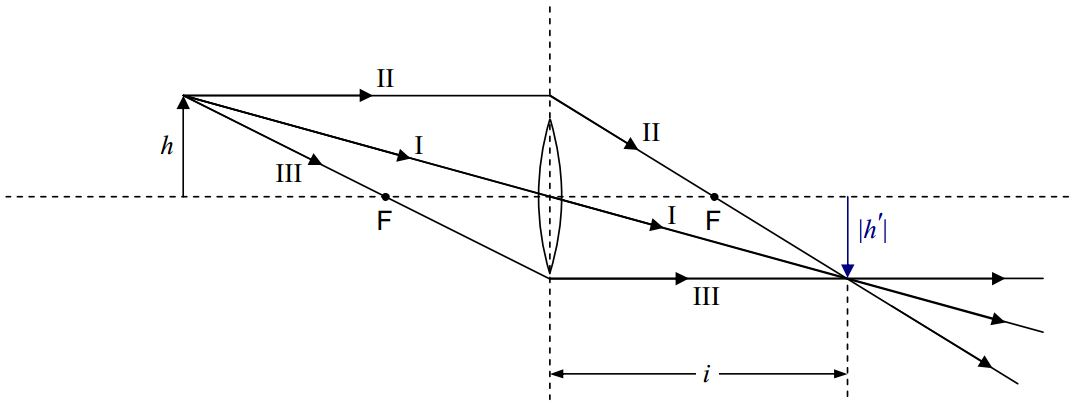in which I have intentionally used a small lens icon to remind you that, in using the principal ray diagram to locate the image, we don’t really care whether or not the principal rays actually hit the lens. Let’s, for the case at hand, consider the diagram to be a life-size diagram of an actual lens. As, important as they are in helping us identify the location of the image, clearly, for the case at hand, Principal Rays II and III do not actually contribute to the image. Principal Ray I does contribute to the image. Let’s draw in some more of the contributors:The fact that every ray that comes from the tip of the object and hits the lens contributes to the image of the tip of the arrow (and the corresponding fact for each and every point on the object) explains why you can cover up a fraction of the lens (such as half the lens) and still get a complete image (albeit dimmer).

## The Power of a Lens

When an ophthalmologist writes a prescription for a spherical lens, she or he will typically write either a value around $$-.5$$ or $$.5$$, or, a value around $$-500$$ or $$500$$ without units. You might well wonder what quantity the given number is a value for, and what the units should be. The answer to the first question is that the physical quantity is the power of the lens being prescribed. In this context, the power is sometimes called the optical power of the lens. The power of a lens has nothing to do with the rate at which energy is being transformed or transferred but instead represents the assignment of a completely different meaning to the same word. In fact, the power of a lens is, by definition, the reciprocal of the focal length of the lens:

$P=\frac{1}{f} \label{29-3}$

In that the SI unit of focal length is the meter (m), the unit of optical power is clearly the reciprocal meter which you can write as $$\frac{1}{m}$$ or $$m^{-1}$$ in accord with your personal preferences. This unit has been assigned a name. It is called the diopter, abbreviated $$D$$. Thus, by definition,

$1D=\frac{1}{m} \nonumber$

Thus, a value of $$-.5$$ on the ophthalmologist’s prescription can be interpreted to mean that what is being prescribed is a lens having a power of $$-0.5$$ diopters. The minus sign means that the lens is a concave (diverging) lens. Taking the reciprocal yields:

$f=\frac{1}{P}=\frac{1}{-0.5 D}=-2 \frac{1}{D}=-2m \nonumber$

If you see a number around $$-500$$ or $$500$$ on the ophthalmologist’s lens prescription, you can assume that the ophthalmologist is giving the power of the lens in units of millidiopters (mD). $$500$$ mD is, of course, equivalent to $$.5D$$. To avoid confusion, if you are given an optical power in units of $$mD$$, convert it to units of diopters before using it to calculate the corresponding focal length.

## Two-Lens Systems

To calculate the image of a two-lens system, one simply calculates the position of the image for the lens that light from the object hits first, and then uses that image as the object for the second lens. In general, one has to be careful to recognize that for the first lens, the object distance and the image distance are both measured relative to the plane of the first lens. Then, for the second lens, the object distance and the image distance are measured relative to the plane of the second lens. That means that, in general, the object distance for the second lens is not equal in value to the image distance for the first lens.

For instance, in the following diagram of two lenses separated by $$12$$cm, if the object is to the left of the first lens, and $$i_1$$ turns out to be $$8$$ cm to the right of the first lens,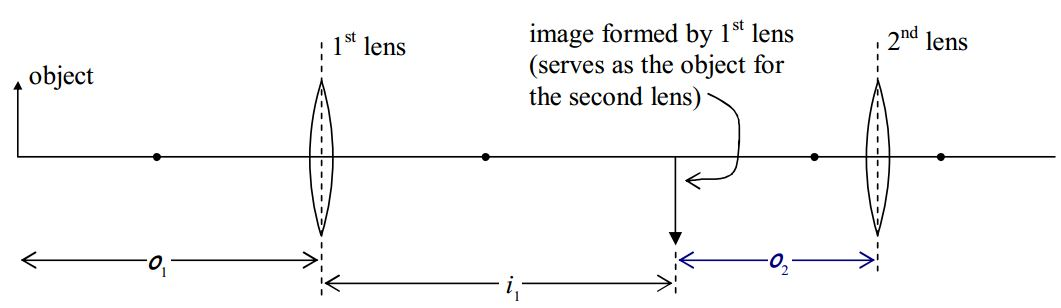then $$o_2$$, the object distance for the second lens, is $$4$$ cm. A peculiar circumstance arises when the second lens is closer to the first lens than the image formed by the first lens is. Suppose for instance, that we have the image depicted above, formed by the first lens: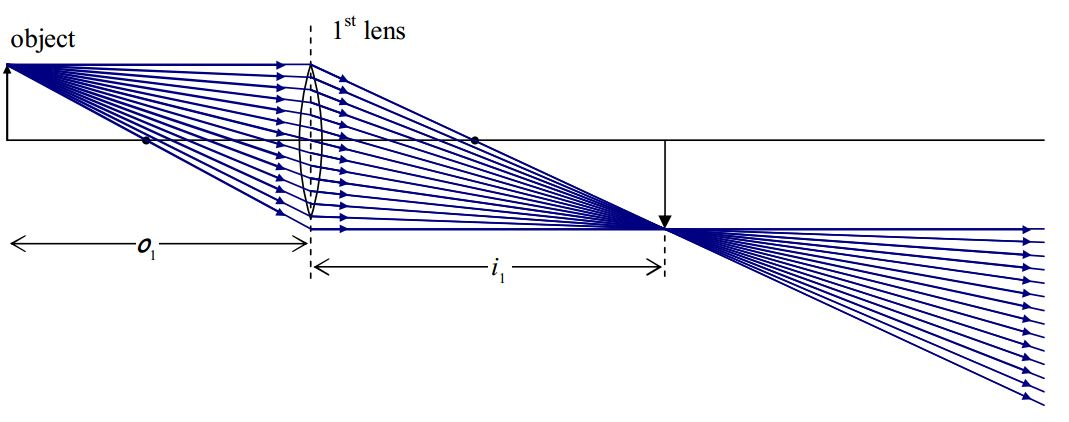Now suppose that we put a second lens in between the 1st lens and the image.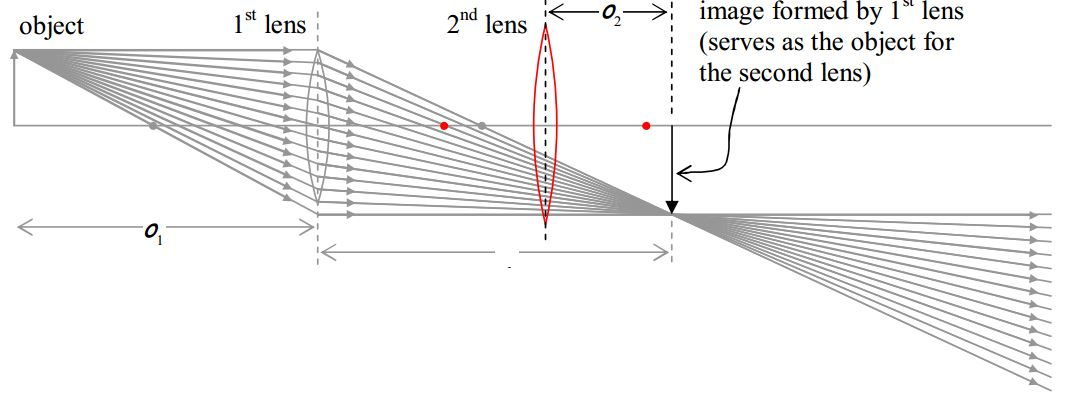Note that, for the second lens, we have an object to the right of the lens, but, the light associated with that object approaches the object from the left! This can only happen when the object is actually an image formed by another lens. In such a case, we call the object a virtual object. More generally, when an object’s light approaches a lens from the side opposite that side to which the object is, the object is considered to be a virtual object, and, the object distance, is, by convention, negative. So, we have one more convention to put in a table for you:

 Physical Quantity Symbol Sign Convention Object Distance $$o$$ + for real object (always the case for a physical object) - for virtual object (only possible if "object" is actually the image formed by another lens)

In forming the ray-tracing diagram for the case of the virtual object, we have to remember that every ray coming into the second lens is headed straight for the tip of the arrow that is the virtual object for the second lens. Thus, our Principal Ray I is one that is headed straight toward the tip of the arrow, and, is headed straight toward the center of the lens. It goes straight through.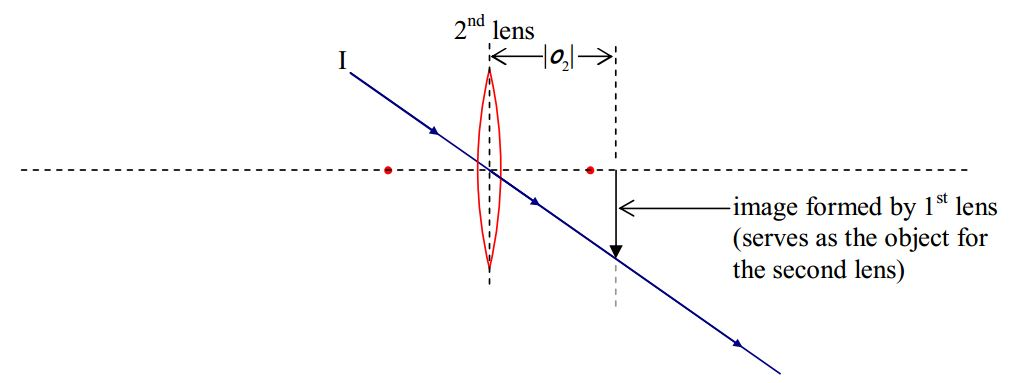Principal Ray II is headed straight for the head of the object along a line that is parallel to the principal axis of the lens. At the plane of the lens it jumps onto the straight line path that takes it straight through the focal point on the other side of the lens.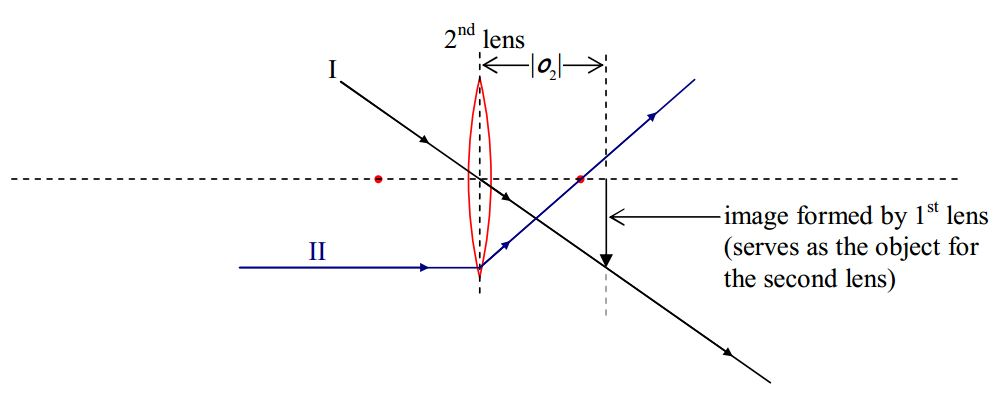Principal Ray III, is headed straight toward the tip of the virtual object, and, on its way to the lens, it passes through the focal point on the side of the lens from which it approaches the lens. When it hits the plane of the lens, Principal Ray III adopts a path that is parallel to the principal axis of the lens.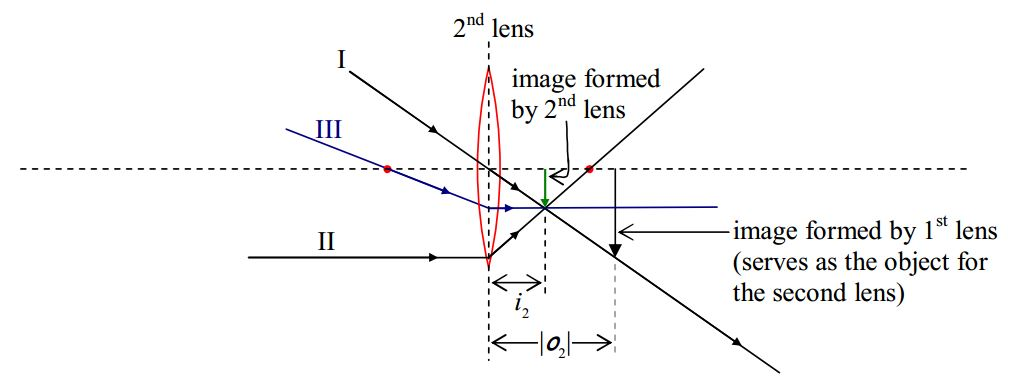Note that, for the case at hand, we get a real image. Relative to the virtual object, the image is not inverted. The virtual object was already upside down. The fact that we can draw a raytracing diagram for the case of a virtual object means that we can identify and analyze similar triangles to establish the relationship between the object distance, the image distance and the focal length of the lens. Doing so, with the convention that the object distance of a virtual object is negative, again yields the lens equation $$\frac{1}{f}=\frac{1}{o}+\frac{1}{i}$$.

Here’s a diagram of the entire two-lens system for the case at hand:Note that the real image of lens 1 alone is never actually formed, but it was crucial in our determination of the image location, orientation, and size, in the case of the two-lens system.

This page titled B29: Thin Lenses - Lens Equation, Optical Power is shared under a CC BY-SA 2.5 license and was authored, remixed, and/or curated by Jeffrey W. Schnick via source content that was edited to the style and standards of the LibreTexts platform; a detailed edit history is available upon request.